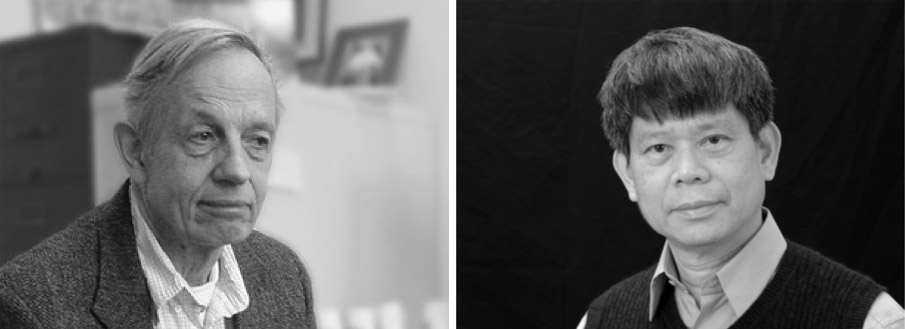## John Nash Bang Yen ChenIn the neighbourhood of any point, any Riemannian manifold M looks like a Euclidean space, such that a Riemannian space might be considered as an infinity of Euclidean spaces which are fitted together in some “sensible” manner. The main difference between Euclidean and general Riemannian spaces lies in the fact that, when parallely moving a rectangular co-ordinate system S from a point p to an arbitrary other point q in M along different curves, the resulting rectangular co-ordinate systems which are then obtained at q do not co-incide, but the one can be obtained from the other by the application of a Euclidean orthogonal transformation at q. From this point of view, the Riemannian spaces can be seen as non-holonomic Euclidean spaces. Yet, by the 1956 isometrical embedding theorem of Nash, every abstract n-dimensional (definite) Riemannian manifold can, in many ways, be considered as a submanifold in Euclidean spaces of significantly high dimensions n+m.

Therefore, Riemannian geometry conceptually essentially is equivalent to the intrinsic geometry of the Riemannian submanifolds of arbitrary dimensions n and co-dimensions m in Euclidean ambient spaces. In this context, the knowledge of fundamental links between the intrinsic and the extrinsic geometries of submanifolds is crucial.
In the 19nineties, Bang-Yen Chen initiated significant advances with his new scalar valued Riemannian curvature invariants, by deriving general optimal inequalities between various intrinsic and extrinsic characteristics of submanifolds. The corresponding Chen and Wintgen ideal submanifolds, i.e. the shapes which are assumed by given abstract Riemannian manifolds when realising them in Euclidean spaces as submanifolds for which the extrinsic tensions are as small as possible, manifest intrinsic symmetry properties of Deszcz type (e.g. Thurston geometries and all physically most relevant spacetimes).